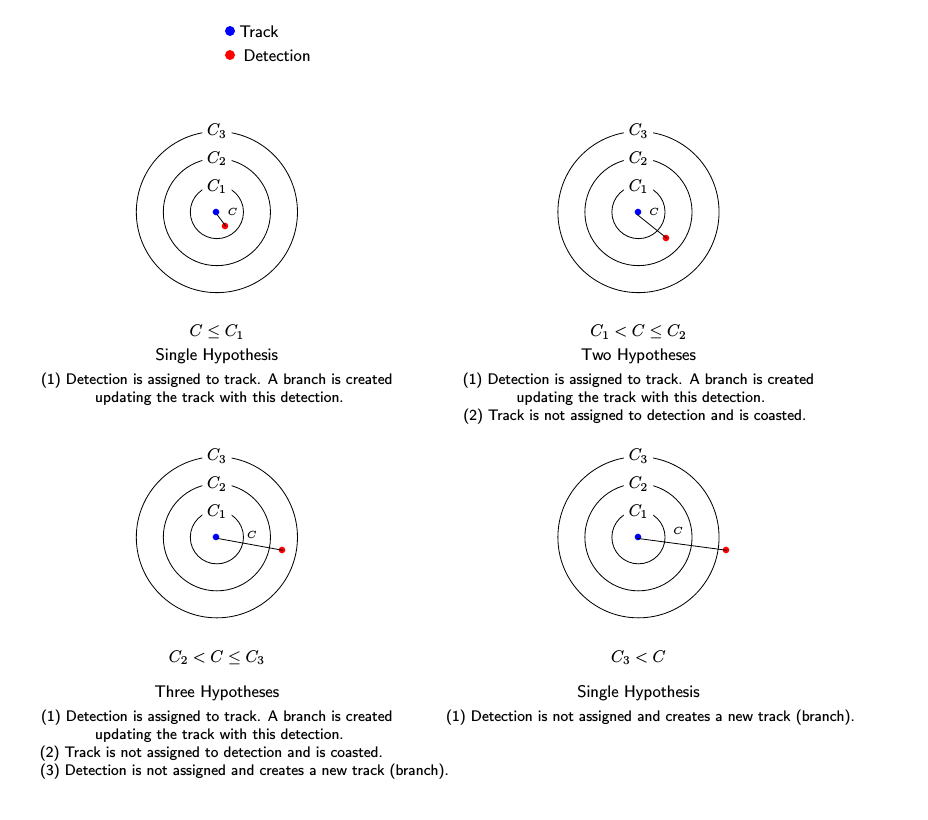# assignTOMHT

Track-oriented multi-hypotheses tracking assignment

## Syntax

``[assignments,unassignedrows,unassignedcolumns] = assignTOMHT(costmatrix,costThreshold)``

## Description

example

````[assignments,unassignedrows,unassignedcolumns] = assignTOMHT(costmatrix,costThreshold)` returns a table of assignments, `assignments`, of detections to tracks using a track-oriented multi-hypothesis algorithm (TOMHT).The cost of each potential assignment is contained in the cost matrix, `costmatrix`. Each matrix entry represents the cost of a possible assignments. Matrix rows represent tracks and columns represent detections. All possible assignments are represented in the cost matrix. The lower the cost, the more likely the assignment is to be made. Each track can be assigned to at most one detection and each detection can be assigned to at most one track. If the number of rows is greater than the number of columns, some tracks are unassigned. If the number of columns is greater than the number of rows, some detections are unassigned. You can set an entry of `costmatrix` to `Inf` to prohibit an assignment.`costThreshold` represents the set of three gates used for assigning detections to tracks.The function returns a list of unassigned tracks, `unassignedrows`, and a list of unassigned detections, `unassignedcolumns`.```

## Examples

collapse all

Find the assignments from a cost matrix using `assignTOMHT` with a nonzero C1 gate and a nonzero C2 gate.

Create a cost matrix that assigns:

• Track 1 to detection 1 within the C1 gate and detection 2 within the C2 gate.

• Track 2 to detection 2 within the C2 gate and detection 3 within the C3 gate.

• Track 3 is unassigned.

• Detection 4 is unassigned.

```costMatrix = [4 9 200 Inf; 300 12 28 Inf; 32 100 210 1000]; costThresh = [5 10 30];```

Calculate the assignments.

`[assignments, unassignedTracks, unassignedDets] = assignTOMHT(costMatrix,costThresh)`
```assignments = 4x2 uint32 matrix 1 1 1 2 2 2 2 3 ```
```unassignedTracks = 2x1 uint32 column vector 2 3 ```
```unassignedDets = 2x1 uint32 column vector 3 4 ```

Tracks that are assigned detections within the C1 gate are not considered as unassigned. For example, track 1. Detections that are assigned to tracks within the C2 gate are not considered as unassigned. For example, detections 1 and 2.

## Input Arguments

collapse all

Cost matrix, specified as an M-by-N matrix. M is the number of tracks to be assigned and N is the number of detections to be assigned. Each entry in the cost matrix contains the cost of a track and detection assignment. The matrix may contain `Inf` entries to indicate that an assignment is prohibited. The cost matrix cannot be a sparse matrix.

Data Types: `single` | `double`

Assignment gates, specified as a positive, real-valued three-element vector `[c1gate,c2gate,c3gate]` where ```c1gate <= c2gate <= c3gate```.

Example: `[0.1,0.3,0.5]`

Data Types: `single` | `double`

## Output Arguments

collapse all

Assignment of detections to track, returned as an integer-valued L-by-2 matrix where L is the number of assignments. The first column of the matrix contains the assigned track indices and the second column contains the assigned detection indices.

Data Types: `uint32`

Indices of unassigned tracks, returned as an integer-valued P-by-1 column vector.

Data Types: `uint32`

Indices of unassigned detections, returned as an integer-valued Q-by-1 column vector.

Data Types: `uint32`

## Algorithms

collapse all

### Assignment Thresholds for Multi-Hypothesis Tracker

Three assignment thresholds, C1 , C2, and C3, control (1) the assignment of a detection to a track, (2) the creation of a new branch from a detection, and (3) the creation of a new branch from an unassigned track. The threshold values must satisfy: C1 <= C2 <= C3.

If the cost of an assignment is `C = costmatrix(i,j)`, the following hypotheses are created based on comparing the cost to the values of the assignment thresholds. Below each comparison, there is a list of the possible hypotheses.Tips:

• Increase the value of C3 if there are detections that should be assigned to tracks but are not. Decrease the value if there are detections that are assigned to tracks they should not be assigned to (too far away).

• Increasing the values C1 and C2 helps control the number of track branches that are created. However, doing so reduces the number of branches (hypotheses) each track has.

• To allow each track to be unassigned, set C1 = 0.

• To allow each detection to be unassigned, set C2 = 0.

 Werthmann, John R. "Step-by-step description of a computationally efficient version of multiple hypothesis tracking." In Signal and Data Processing of Small Targets 1992, vol. 1698, pp. 288-300. International Society for Optics and Photonics, 1992.

##### Support평가판 신청